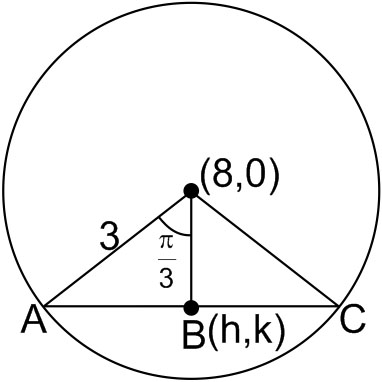#### Letbe the circle with centre (0, 0) and radius 3 units. The equation of the locus of the mid points of chord of the circlethat subtend an angle ofat its centre is Option 1)Option 2)Option 3)Option 4)As we learnt in

Equation of a circle -- wherein

Circle with centreand radius.Inh2 + k2 = 9/4

Option 1)This is incorrect option

Option 2)This is incorrect option

Option 3)This is incorrect option

Option 4)This is correct option

##### Posted by